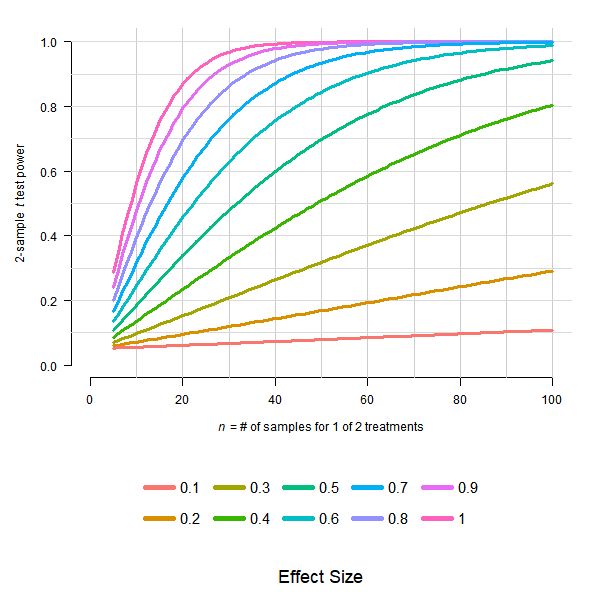Explore our sister journals here.

Maintenance Notice

Due to necessary scheduled maintenance, the JMIR Publications website will be unavailable from Wednesday, July 01, 2020 at 8:00 PM to 10:00 PM EST. We apologize in advance for any inconvenience this may cause you.

Who will be affected?

Right click to copy or hit: ctrl+c (cmd+c on mac)

## Comment on “Designing Robust N-of-1 Studies for Precision Medicine: Simulation Study and Design Recommendations”

Department of Biostatistics, University of Arkansas for Medical Sciences, Little Rock, AR, United States

### Corresponding Author:

Reid D Landes, PhD

Department of Biostatistics

University of Arkansas for Medical Sciences

4301 W Markham St

Slot 781

Little Rock, AR, 72205

United States

Phone: 1 501 526 6714

Email: rdlandes@uams.edu

Related Article:

#### J Med Internet Res 2020;22(9):e16179

doi:10.2196/16179

### KEYWORDS

In “Designing robust N-of-1 studies for precision medicine: Simulation study and design recommendations” by Percha et al , the authors use misleading language when speaking about the required numbers of samples regarding results in Figure 4a. For example, they write on page 8:

In Figure 4a, we see that for effect sizes of 0.1, 0.2, and 0.3, more than 100 samples are needed to obtain a power of 0.8 (at a standard 5% significance level). For an effect size of 0.4, at least 100 samples are needed. For effect sizes of 0.5, 0.6, 0.7, 0.8, 0.9, and 1.0, the numbers of samples needed to attain a power of 0.8 are approximately 65, 45, 35, 26, 21, and 18, respectively. [Figure 4]

Since Figure 4a is exactly equivalent to power curves from a two-sample, equal-variance t test (see Figure 1; generating R code provided in Textbox 1), the numbers of samples are for one of the two treatments; thus, the total numbers of samples are doubled. An easy fix in most instances of the unclear language is to add “per treatment” after “samples.” I provide a list of potential clarifying edits to the article’s text below (but may have missed some instances):

• Figure 4c caption: “(ie, number of samples per treatment, with sampling rate fixed at 1 sample per time unit)”
• Figure 4a and 4b: the label for the horizontal axis should be “Number of samples per treatment
• Page 8: “In Figure 4a, we see that for effect sizes of 0.1, 0.2, and 0.3, more than 100 samples per treatment are needed to obtain a power of 0.8 (at a standard 5% significance level). For an effect size of 0.4, at least 100 samples per treatment are needed. For effect sizes of 0.5, 0.6, 0.7, 0.8, 0.9, and 1.0, the numbers of samples per treatment needed to attain a power of 0.8 are approximately 65, 45, 35, 26, 21, and 18, respectively.”
• Page 9: “For an effect size of 0.5 and σp=0.0, 0.4, 0.8, 1.2, 1.6, 2.0, the numbers of samples per treatment needed to obtain a power of 0.8 are 61, 76, 89, 111, 135, and 176, respectively. For an effect size of 1.0, the numbers of samples per treatment needed are 20, 24, 28, 34, 43, and 53, respectively.”
• Page 9: “For σp=0.0, 0.4, 0.8, 1.2, 1.6, 2.0 and α=0.1, the numbers of samples per treatment required are 36, 64, 110, 174, 228, and 250, respectively. For α=10.0, the numbers of samples per treatment required are only 20, 23, 28, 34, 42, and 53, respectively.”Figure 1. For effect sizes ranging from 0.1 to 1.0, power of a 0.05 level two-sample t test plotted by n, the number of samples in one treatment group. Total sample size is assumed to be 2n.Textbox 1. R code.

Percha and colleagues have agreed to the above changes; these changes have been made to the original paper.

#### Conflicts of Interest

None declared.

1. Percha B, Baskerville EB, Johnson M, Dudley JT, Zimmerman N. Designing Robust N-of-1 Studies for Precision Medicine: Simulation Study and Design Recommendations. J Med Internet Res 2019 Apr 01;21(4):e12641 [FREE Full text] [CrossRef] [Medline]